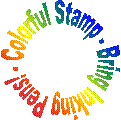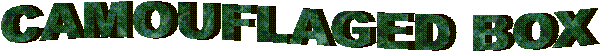Mathman 21 - WOOT! Bryan Henne, Kyle Zimmermann, and John PaustianTerrain: Grassy park Difficulty: Depends on math ability Placed by: The Dragon (Mathman) and his Honors Advanced Algebra students of 2005-06 Location: ??? Park in Germantown, WI County: Washington, WI Materials needed: Calculator, Compass, Writing Utensil, Personal Stamp, Inking PensStudents and parents of students should read my introduction to letterboxing before seeking the boxes.

These clues rely on the use of matrices to encode/decode clues.  Go to this page to learn how to do this on a free graphics calculator simulator or to do it by hand.

Be sure to read through all the clues before looking for the boxes so you know what is expected of you.

Clues:

First off, decode the following message to know the park to go to:Now that you have found the location of the box, drive to the park. As you enter the park turn into the left parking spot and park next to the green dumpster.

Go down to the jungle gym and have a swing on the monkey bars. Count how many bars there are (x = ____)  Now if y varies directly as x, y=kx, plug in the number of bars for x and 165 for y, and figure out the constant of variation. This number will be very helpful later when trying to find where the box is.

Now go up to the hexagon shaped platform and stand in the middle of it. Stand facing in between the two slides and find the discriminant of this equation:  Y = 3x² + 4x - 6. Now if you think this equation has real roots then go down the right slide. If you think it has imaginary roots go down the left slide.

From the end of the correct slide you should see a path a little ways ahead of you. Go down the path to the first intersection. Take the path whose bearing is the discriminant you found above.  Now at the next intersection, go to the black single stripe pole and look at it facing north.  Figure out the roots for this equation:  x² - 180x + 7316   The sum of the roots will be the number of degrees you need to turn to the right. Continue down the path ahead of you until you come to the next intersection (it will be a while and you'll cross a bridge).

At the next intersection, stand in the middle and face so there is a path on your left, right, and straight ahead.
Let f(x) = 2x + 9 and g(x) = 5x - 3.5
Simplify f(x) + g(x) = ______________

Take the coefficient of x and flip it upside down. Then flip it right to left. Your result should look like the first letter of the word for which patch you should take. Follow that path for a while, turning a corner along the way, to the next major intersection.

As you approach the intersection, keep facing the way you are, but stop when you are lined up with the red single striped pole to your right.
FOIL the following to get a quadratic expression:  (2x + 8)(6x + 3) = __________________
Add up all the numbers (coefficients) in the newly formed quadratic expression, and turn that many degrees to your right and proceed down the path now before you.

 As your approach the next intersection, you will see that there are four paths. Now pretend that the intersection of all four paths is the origin on a piece of graph paper. Stand at the origin and turn to the west. You will be facing a green single striped pole. What quadrant is the vertex of f(x) = 5(x + 12)² + 3 in?  This will be the quadrant that the box is in (remember the four paths break up the land to form the four quadrants). Now find the roots of this quadratic equation: x² - 2x - 143 = 0. Pretend the box is at a point in the quadrant that you found in the previous clue.  Use the roots that you just found to make up an ordered pair that would be in that quadrant.  Remembering that you are at point (0,0) go to the point you just found letting one unit be one step. WestEast

Now think all the way back when you found the constant of variation.  The constant of variation will be the number of trunks the tree has (they should all be connected but some may look broken and are lying on the ground). The trunks form a pit  The box is located in the pit under the roots/base of one of the standing trunks and covered by leaves. When you are finished with the box, please re-hide it the way you found it.  Thanks!

Before you set out read the waiver of responsibility and disclaimer.

LbNA Home